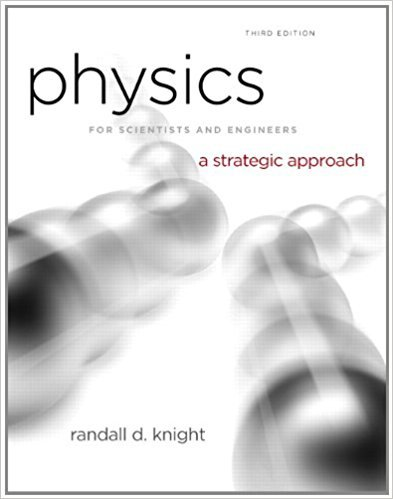×
Get Full Access to Physics For Scientists And Engineers: A Strategic Approach With Modern Physics - 3 Edition - Chapter 17 - Problem 45p
Get Full Access to Physics For Scientists And Engineers: A Strategic Approach With Modern Physics - 3 Edition - Chapter 17 - Problem 45p

×

# An experiment measures the temperature of a 500 gISBN: 9780321740908 69

## Solution for problem 45P Chapter 17

Physics for Scientists and Engineers: A Strategic Approach with Modern Physics | 3rd Edition

• Textbook Solutions
• 2901 Step-by-step solutions solved by professors and subject experts
• Get 24/7 help from StudySoup virtual teaching assistantsPhysics for Scientists and Engineers: A Strategic Approach with Modern Physics | 3rd Edition

4 5 1 301 Reviews
23
3
Problem 45P

Problem 45P

An experiment measures the temperature of a 500 g substance while steadily supplying heat to it. FIGURE shows the results of the experiment. What are the (a) specific heat of the solid phase, (b) specific heat of the liquid phase, (c) melting and boiling temperatures, and (d) heats of fusion and vaporization?

FIGUREStep-by-Step Solution:

Solution 45 P

Step 1 of 5

We are required to calculate the specific heat of the solid phase

We are required to calculate the specific heat of the liquid phase

We need to identify the melting and boiling points temperatures

We have to calculate  heat of fusion and  heat of vaporization

Step 2 of 5

Step 3 of 5

##### ISBN: 9780321740908

This full solution covers the following key subjects: heat, figure, specific, phase, experiment. This expansive textbook survival guide covers 17 chapters, and 1439 solutions. Physics for Scientists and Engineers: A Strategic Approach with Modern Physics was written by and is associated to the ISBN: 9780321740908. Since the solution to 45P from 17 chapter was answered, more than 2548 students have viewed the full step-by-step answer. The full step-by-step solution to problem: 45P from chapter: 17 was answered by , our top Physics solution expert on 08/30/17, 04:34AM. The answer to “An experiment measures the temperature of a 500 g substance while steadily supplying heat to it. FIGURE shows the results of the experiment. What are the (a) specific heat of the solid phase, (b) specific heat of the liquid phase, (c) melting and boiling temperatures, and (d) heats of fusion and vaporization?FIGURE” is broken down into a number of easy to follow steps, and 52 words. This textbook survival guide was created for the textbook: Physics for Scientists and Engineers: A Strategic Approach with Modern Physics, edition: 3.

Unlock Textbook Solution